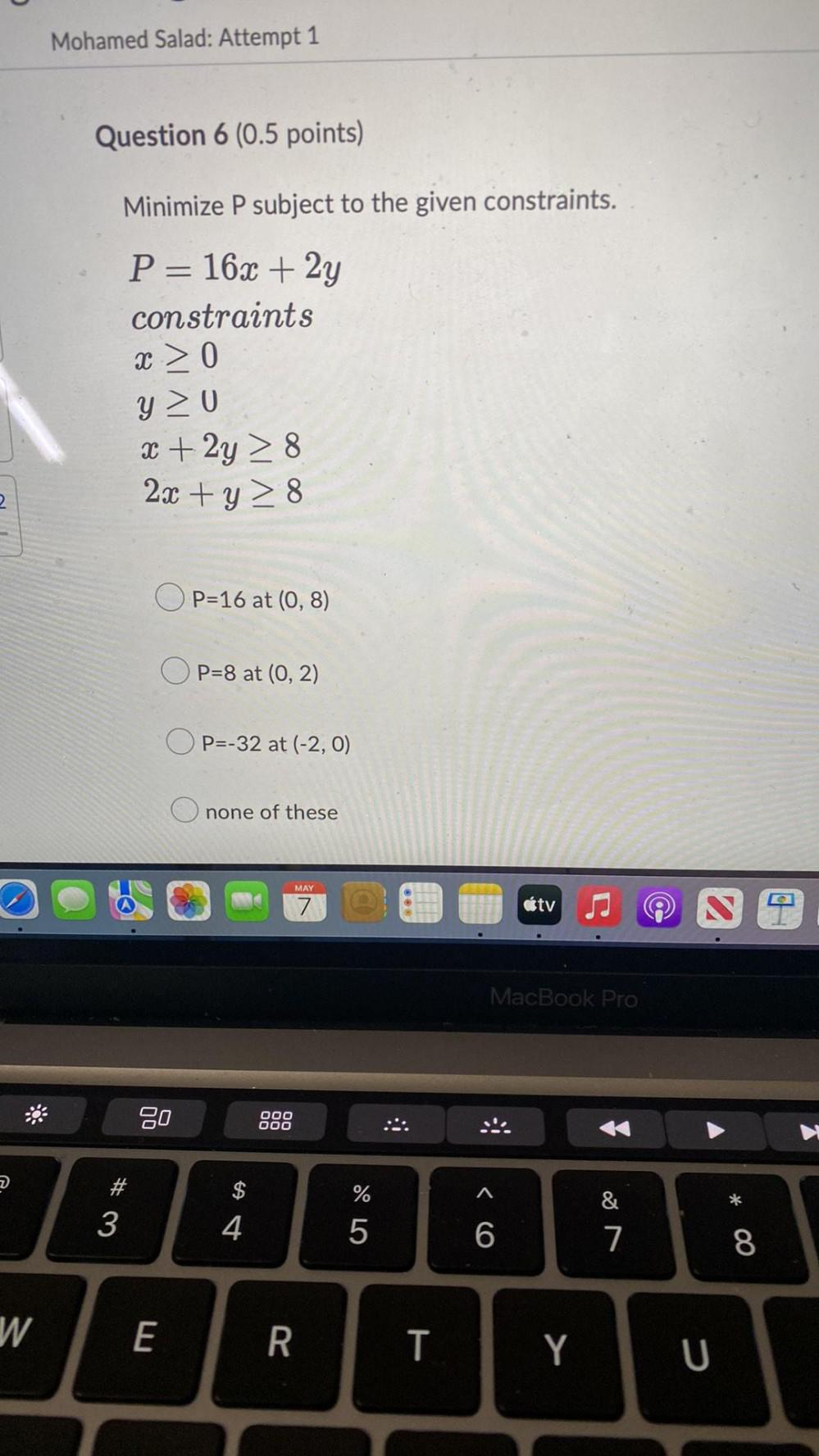Question:

# Minimize P subject to the given constraints. P = 16x + 2y constraints x ≥ 0 y ≥ 0 x + 2y ≥ 8 2x + y ≥ 8 a. P =16 at (0, 8) b. P = 8 at (0, 2) c. P = -32 at (-2, 0) d. none of theseMinimize P subject to the given constraints. P = 16x + 2y constraints x ≥ 0 y ≥ 0 x + 2y ≥ 8 2x + y ≥ 8 a. P =16 at (0, 8) b. P = 8 at (0, 2) c. P = -32 at (-2, 0) d. none of these